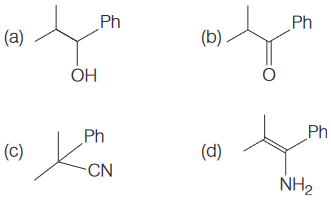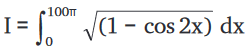### Timezupp!!

-- : --
Are you sure?
Question 1 reset

Temperature of an ideal gas, initially at 27° C, is raised by 6° C. The rms velocity of the gas molecules will

Question 2 reset

When the frequency of the AC voltage applied to a series LCR circuit is gradually increased from a low value, the impedance of the circuit

Question 3 reset

A bullet of mass 4.2 × 10−2 kg, moving at a speed of 300 ms−1, gets stuck into a block with a mass 9 times that of the bullet. If the block is free to move without any kind of friction, the heat generated in the process will be

Question 4 reset
When a semiconducting device is connected in series with a battery and a resistance, a current is found to flow in the circuit. If however, the polarity of the battery is reversed, practically no current flows in the circuit. The device may be
Question 5 reset

In the circuit given below, the binary inputs at A and B are both 1 in one case and both 0 in the next case. The respective outputs at Y in these two cases will beQuestion 6 reset

A block of mass 1 kg starts from rest at x = 0 and moves along the X-axis under the action of a force F = kt, where t is time and k = 1 Ns−1. The distance the block will travel in 6 seconds is

Question 7 reset

Consider the circuit given below. The potential difference VBC between the points B and C isQuestion 8 reset

Directions: One or more answer(s) is (are) correct.

Two long parallel wires separated by 0.1 m carry currents of 1 A and 2 A, respectively in opposite directions. A third current-carrying wire parallel to both of them is placed in the same plane such that it feels no net magnetic force. It is placed at a distance of

Question 9 reset

Which of the following has the dimension of [ML0T-2] ?

Question 10 reset

Dissolving NaCN in de-ionised water will result in a solution having

Question 11 reset

Which of the following atoms should have the highest Ist electron affinity?

Question 12 reset

The correct order of acid strengths of benzoic acid ( X ), peroxybenzoic acid ( Y ) and p-nitrobenzoic acid ( Z ) is

Question 13 reset
The yield of acetanilide in the reaction (100% conversion) of 2 moles of aniline with 1 mole of acetic anhydride is
Question 14 reset
ADP and ATP differ in the number of
Question 15 reset

For the reaction given belowthe structure of the product Q isQuestion 16 reset

The equation of the plane through (1, 2, -3) and (2, -2, 1) and parallel to X-axis is

Question 17 reset

If p, q are odd integers, then the roots of the equation 2px2 + (2p + q)x +q = 0 are

Question 18 reset

The focus of the conic x2 - 6x + 4y + 1= 0 is

Question 19 resetQuestion 20 reset

Find the value of I if,## Time Over

Please wait while we are submitting your test

Are you sure you want to finish test early?